## Basic geometry: language and labels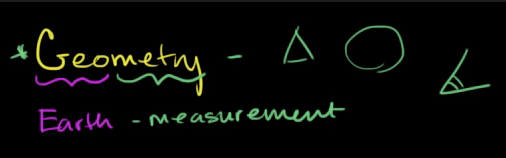Notes from Khan Academy video Basic geometry: language and labels | Introduction to Euclidean geometry | Geometry | Khan Academy

• Word geometry is made up of 2 components: "geo" and "metry":
• "Geo" - earth
• "metry" - measurement
• Therefore, geometery is about how shapes and space (things we see) related to each other.

## Plane

• A plane is a flat surface that has 2 dimensions: width and height and goes on infinity in either direction.

## Point

• A point refers to a dot on that plane and is the most basic geometric idea.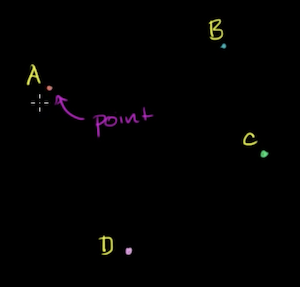• points are commonly labelled using upper case letters: A, B, C, D etc

• A point is said to be 0-dimension: it doesn't provide any direction information.

## Line Segment

• A line that connects 2 points, is refered to as a line segment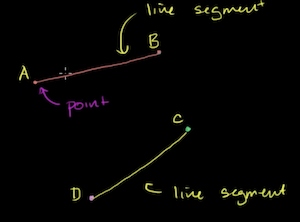• A line segment is described using its endpoints: the points that it is connected by.
• In math notation, a line is drawn over the endpoints to denote that it's a line segment.

$\overline{AB}$

• The endpoints of a line segment can be inversed:

$\overline{AB} = \overline{BA}$

• A line segment is one-dimension - you can move back and forth along it.

## Ray

• A line that starts from one point that continues on infinitely in one direction is called a Ray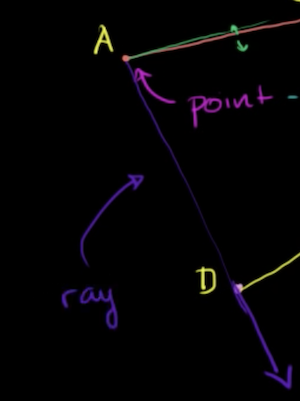• The order of a ray is meaninful, unlike a line segment, as it describes the direction of the ray:

$\overrightarrow{AB} \neq \overrightarrow{BA}$

• The starting point is referred to the endpoint

## Line

• A line that continues infinitely in either direction is formally called a line.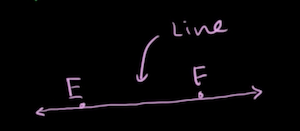• A line is described by the endpoints it intersects, typically in alphabetical order though the order does not matter.

$\overleftrightarrow{AB} = \overleftrightarrow{BA}$

## Collinear points

• If you have a line segment and introduce a point in the middle, you could describe all 3 points as "collinear" - they sit on the same line.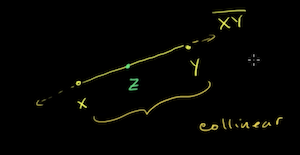• In the above picture, if the distance between $XZ$ was the same as the distance between $ZX$, then you would refer to Z as the midpoint of the line segment $XY$.